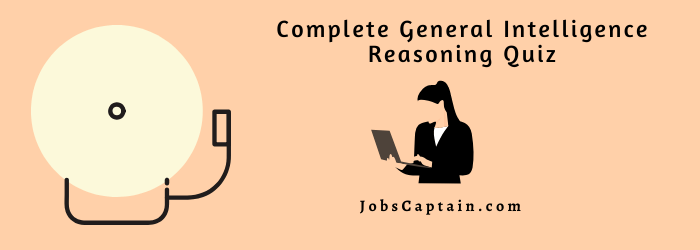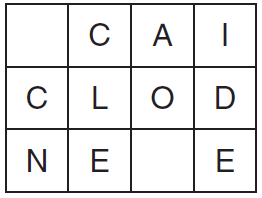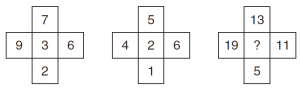# General Intelligence Reasoning Quiz

Online Quiz Questions Answers with Detailed Explanation for Reasoning & General Intelligence Materials pdf for All Competitive Exams Like GPSC, UPSC, SSC, RRB, Defence, Clerk and all State PSC Exams.

Evaluate your Basic Knowledge of reasoning skills by trying the below online General Intelligence question-answer quiz.

Question 1: Which group of letters is the odd one out?

 CEFH LNOQ UWXZ HJKN PRSU DFGI

(A) DFGI

(B) PRSU

(C) CEFH

(D) HJKN

(D) HJKN
Explanation
HJKN: it follows the pattern HiJKlmN; the rest follow the pattern CdEFgH

Question 2: Spell out a 12-letter word by moving from letter to adjacent letter, horizontally and vertically (but not diagonally). You must find the starting point and provide the missing letters.(A) Construction

(B) Encyclopedia

(D) Applications

(B) Encyclopedia

Question 3: What numbers should replace the question marks?
100, 95, ?, 79, 68, 55, 40, 23

(A) 88

(B) 82

(C) 72

(D) 73

(A) 88
Explanation
deduct 5, 7, 9, 11, 13, 15, 17

Question 4: What number should replace the question mark?(A) 6

(B) 5

(C) 4

(D) 7

(A) 6
Explanation
(19 + 11) / 5 = 6;  (13 + 5)/ 3 = 6

Question 5: What letter is three to the right of the letter immediately to the left of the letter which is four to the left of the letter G?

 A B C D E F G H

(A) E

(B) H

(C) C

(D) B

(D) B
Explanation
The rectangle increases in size and rotates 90. The square reduces in size and goes inside the figure originally inside the square, which in turn goes inside the rectangle having rotated 180.

Question 6: What comes next?

January
February
April
July
November
April
?????

(A) December

(B) March

(C) October

(D) February

(C) October

Question 7: In the following questions, a series is given with one term missing. Choose the correct alternative from the given ones that will complete the series.

(A) GMSYE

(B) FMSYE

(C) GNSYD

(D) FMYES

(A) GMSYE

Question 8: Select the related word/letters/numbers from the given alternatives:
4 : 20 : : 8 : ?

(A) 74

(B) 70

(C) 72

(D) 78

(C) 72
Explanation
4 × 5 = 20 And 8 × 9 = 72

Question 9: M is son of P, Q is the grand-daughter of O, who is the husband of P. How is M related to O?

(A) Son

(B) Daughter

(C) Mother

(D) Father

(A) Son

Question 10: If “A” means “subtraction”, “B” means “division”, “C” means “addition” and “D” means “multiplication”,

then 305 B 5 A 28 C 43 D 12 = ___________

(A) 569

(B) 549

(C) 560

(D) 530

(B) 549
Explanation
⇒ 305 ÷ 5 – 28 + 43 × 12
⇒ 61 – 28 + 516
⇒ 577 – 28
⇒ 549

Question 11: Select the related word/number from the given alternatives:

CLOSE : DNRWJ : : OPEN : __________

(A) PRHR

(B) PRJQ

(C) RPJB

(D) RZWR

(A) PRHR
Explanation
The 1st, 2nd, 3rd, 4th and 5th letters of the first group are moved one, two, three, four and five steps forward respectively to obtain the corresponding letters of the second group.

Question 12: Select the word which can be formed from the word IMMEDIATELY.

(A) DIALECT

(B) LIMITED

(C) DIAMETER

(D) DICTATE

(B) LIMITED
Explanation
Only the word ‘LIMITED’ can be formed from the original word.

Question 13: Introducing a girl, Ankit says, “She is the sister of the son of my mother’s sister”. How is the girl related to Ankit?

(A) Niece

(B) Daughter

(C) Sister

(D) Cousin

(D) Cousin

Question 14: It was Sunday on jan 2, 2006, what was the day of the week Jan 1, 2010 ?

(A) Sunday

(B) Thursday

(C) Friday

(D) Saturday

(B) Thursday
Explanation

On 31 Dec 2005 it was Friday Number of odd days from the year 2006 to the year 2009 = (1+ 1 + 2 + 1) = 5 days

∴ on 31st Dec 2009, it was = Wednesday, Thus on 1st + Jan 2010 it is Thursday.

Question 15: Neha walks 30m towards south, then turning to her right she walks 30m, then turning to her left, she walks 20m, again she turns to her left and walks 30m. How far is she from her initial position?

(A) 20 mtr

(B) 30 mtr

(C) 50 mtr

(D) 60 mtr

(C) 50 mtr

Question 16: If in a code GONE is written as ILPB then how may CRIB be written in that code?

(A) EUKY

(B) EKUY

(C) EYUK

(D) EOKY

(D) EOKY

Question 17: One morning, Ketan walked towards the sun. After some time he turned left and again to his left. Which direction is he facing?

(A) North

(B) South

(C) East

(D) West

(D) West

Question 18: If India is coded as 27924 and cricket is coded as 1621835 then DIRT will be coded as ___________ .

(A) 9878

(B) 9825

(C) 9165

(D) 9265

(D) 9265

Question 19: If ‘Aman’ = 4, ‘Shivam’ = 6, ‘Science’ = 7, Then ‘Bhim’ = ?

(A) 4

(B) 3

(C) 6

(D) 5

(A) 4
Explanation
Number of letters in the word = 4

Question 20: If “A” denotes “added to”, “B” denotes “divided by”, “C” denotes “multiplied by” and “D” denotes “subtracted from”, then 87 B 3 C 4 A 4 D 50 = ?

(A) 65

(B) 75

(C) 70

(D) 80

(C) 70
Explanation

87 B 3 C 4 A 4 D 50
⇒ 87 ÷ 3 × 4 + 4 – 50
⇒ 29 × 4 + 4 – 50
⇒ 116 + 4 – 50
⇒ 120 – 50
⇒ 70

Question 21: In a certain code language “feel free to fly” = “4l 4e 2o 3y”. “Why the statement” = “3y 3e 9t” then “Media” is coded as _________ .

(A) 5m

(B) 5e

(C) 5a

(D) 4a

(C) 5a
Explanation

Number of alphabet, last letter

Question 22: Mohini is taller than Nita but not shorter than Sarita. Sarita and Mahek are of same height. Mohini is shorter that Hemali. Among them, who is the second tallest?

(A) Mohini

(B) Nita

(C) Hemali

(D) None of the above

(D) None of the above
Explanation

Mahek = Sarita > Mohini > Nita
Hema > Mohini
Second tallest can’t be determined

Question 23: In a certain code language, “CAB” is written as “6” and “LEG” is written as “6”. How is “MAP” written in that code language?

(A) 6

(B) 4

(C) 3

(D) 8

(C) 3
Explanation

CAB = 3 + 1 + 2 = 6
LEG = 12 + 5 + 7 = 24 = 2 + 4 = 6
MAP = 13 + 1 + 16 = 30 = 3 + 0 = 3

Question 24: If 11 # 2 @ 6 = 78 and 15 # 4 @ 8 = 152, then 17 # 6 @ 7 = ?

(A) 161

(B) 143

(C) 221

(D) 157

(A) 161
Explanation

(11 + 2) × 6 = 78
(15 + 4) × 8 = 152
(17 + 6) × 7 = 161

Question 25: A series is given with one term missing. Choose the correct alternative from the given ones that will complete the series.

MN, PQ, TU, YZ, ?

(A) YZ

(B) AB

(C) EF

(D) EJ

(C) EF

Question 26: . In the following question, select the odd word pair from the given alternatives.

(A) Zinc – Metal

(B) Aluminum – Metal

(C) Crocodile – Water

(D) Gold – Metal

(C) Crocodile – Water
Explanation

Crocodile is not water.

Question 27: Select the related number from the given alternatives.

97 : 63 : : 67 : ?

(A) 38

(B) 56

(C) 42

(D) 45

(C) 42
Explanation

9 × 7 = 63
6 × 7 = 42

Question 28: Jayesh is facing towards the east. He turns 270 degrees clockwise and then takes a right turn. Finally, he turns 90 degrees anticlockwise. Which direction is he facing now?

(A) West

(B) South

(C) North

(D) East

(C) North

Question 29: Which set of letters when sequentially placed at the gaps in the given letter series shall complete it?

p_rt_rr_p_rt

(A) rptr

(B) rrtp

(C) ptrr

(D) tprt

(A) rptr
Explanation

prrt/prrt/prrt

Question 30: In the following question, select the odd number pair from the given alternatives.

(A) 17 – 12

(B) 29 – 14

(C) 21 – 16

(D) 31 – 26